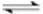Try out our new practice tests completely free!Solved

# Which of the Following Best Represents the Autoionization,or Self-Ionization,of Water

Question 7
Multiple Choice
Multiple Choice

## Which of the following best represents the autoionization,or self-ionization,of water?

A)H2O(l)→ H+(aq)+ OH-(aq)
B)2H2O(l)2H2(aq)+ O2(aq)
C)2H2O(l)H3O+(aq)+ OH-(aq)
D)H2O(l)→ H2(aq)+ O2-(aq)
E)H+(aq)+ OH-(aq)→ H2O(l)

Choose question tag10+ million students use Quizplus to study and prepare for their homework, quizzes and exams through 20m+ questions in 300k quizzes.

### Chemistry

Explore our library and get Biochemistry Homework Help with various study sets and a huge amount of quizzes and questions

Get Free Access Now!Textbook Solutions

Find all the solutions to your textbooks, reveal answers you would’t find elsewhereSearch By Image

Scan any paper and upload it to find exam solutions and many moreFlashcards

Studying is made a lot easier and more fun with our online flashcards